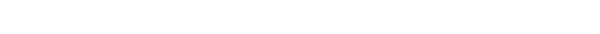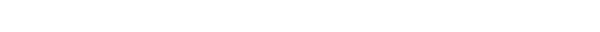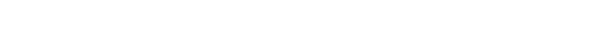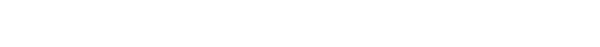### 一 统计学基础运算

#### 1 方差的计算

A组 = [50,60,40,30,70,50] B组 = [40,30,40,40,100]#### 2 使用numpy计算标准差和方差

```import numpy as np
# 创建一个二维数组
arr = np.array([[3, 7, 25, 8, 15, 20],
[4, 5, 6, 9, 14, 21]])
# 计算方差
print(arr.var())
print(np.var(arr))
# 计算标准差
print(arr.std())
print(np.std(arr))
# 计算轴0方向方差
print(arr.var(axis=0))
print(np.var(arr, axis=0))
# 计算轴1方向方差
print(arr.var(axis=1))
print(np.var(arr, axis=1))
# 计算轴0方向标准差
print(arr.std(axis=0))
print(np.std(arr, axis=0))
# 计算轴1方向标准差
print(arr.std(axis=1))
print(np.std(arr, axis=1))```

### 二 二项式定理

#### 1 二项式系数2ab这一项可以用排列组合的知识来理解，从(a+b)(a+b)分别选出a和b的可能性，那幺一共有两种情况：#### 2 用Python获得二项系数

```import itertools
import numpy as np
# 等待排列的数组
arr = [1, 2, 3, 4, 5]
# 排列的实现P
print(list(itertools.permutations(arr, 3)))
# 组合的实现C
print(list(itertools.combinations(arr, 3)))

# 获取二项系数的函数
# 支持两个参数，第一个是n，第二个是k
def get_binomial_coefficient(n, k):
return len(list(itertools.combinations(np.arange(n), k)))

print(get_binomial_coefficient(3, 1))```### 三 独立实验与重复实验

P(A∩B) = P(A) * P(B)P(A∪B)=P(A)+P(B)```import itertools
import numpy as np
"""

"""

# 声明一个函数来求组合问题
def get_binomial_coefficient(n, k):
return len(list(itertools.combinations(np.arange(n), k)))

# 每题答对的概率
P_true = 1 / 4
# 每题答错的概率
P_false = 3 / 4
# 求答对0到5题的组合情况
# 答对0题的组合情况
zero = get_binomial_coefficient(5, 0)
# 答对1题的组合情况
one = get_binomial_coefficient(5, 1)
# 答对2题的组合情况
two = get_binomial_coefficient(5, 2)
# 答对3题的组合情况
three = get_binomial_coefficient(5, 3)
# 答对4题的组合情况
four = get_binomial_coefficient(5, 4)
# 答对5题的组合情况
five = get_binomial_coefficient(5, 5)
# # # 答对一半以上的概率(答对3题、4题、5题)
last = three * pow(P_true, 3) * pow(P_false, 2) + four * pow(P_true, 4) * pow(P_false, 1) + five * pow(P_true, 5) * pow(
P_false, 0)
print(last)```

### 四 ∑符号及其意义Σ(读西格玛)符号在数学中非常的常见，在以后的学习中，你也几乎可以在任意一个算法模型中见到这个符号，所以它的特点也一定要掌握。

Σ可以使用分配率，我们一起来看一个例子：Σ的计算公式我们暂时先学习这两个，其他的公式后边用到的时候我们再来结合着场景进行学习。

### 六 伯努利分布

P(x=1) = p ， P(x=0) = 1-p

```# 导入 matplotlib库，用来画图，关于画图我们后边会有专门的章节进行讲解
import matplotlib.pyplot as plt
# 导入numpy
import numpy as np
n_person = 200
n_times = 500
t = np.arange(n_times)
# 创建包含1和-1两种类型元素的随机数组来表示输赢
# *2 -1 是为了随机出1 和-1，(n_person, n_times)表示生成一个200*500的二维数组
steps = 2 * np.random.random_integers(0, 1, (n_person, n_times)) - 1
# 计算每一组的输赢总额
amount = np.cumsum(steps, axis=1)
# 计算平方
sd_amount = amount ** 2
# 计算所有参加赌博的组的平均值
average_amount = np.sqrt(sd_amount.mean(axis=0))
print(average_amount)
# 画出数据,用绿色表示，并画出平方根的曲线，用红色表示
plt.plot(t, average_amount, 'g.', t, np.sqrt(t), 'r')
plt.show()```

### 七 二项分布### 八 条件概率

P(A|B) = P(A∩B)÷P(B)

P(B|A) = P(A∩B)÷P(A)

P(A∩B) = P(B) * P(A|B)

P(A∩B) = P(A) * P(B|A)

P(A|B) = P(B|A) * P(A) / P(B)

### 九 全概率

P(A)=P(A|B1)P(B1)+P(A|B2)P(B21)+P(A|B3)P(B3)+P(A|B4)P(B4)

**以上这就是全概率公式和他的推导过程。

### 十 贝叶斯定理1. 根据简单贝叶斯公式来进行计算一下，也就是P(病|阳性)=P(阳性|病)P(病)/P(阳性)。

1. 进一步的把P(阳性)换算成全概率公式P(阳性)=P(病)P(阳性|病)+P(健康)P(阳性|健康)。

1. 最终得到P(病|阳性)=P(阳性|病)P(病)/(P(病)P(阳性|病)+P(健康)P(阳性|健康))

P(病|阳性) = 1 * 0.001 / (0.001 * 1 + 0.999 * 0.05) = 0.0196 = 1.96%